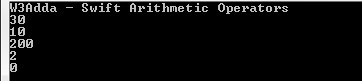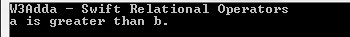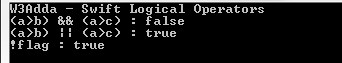# Swift Operators

In this tutorial you will learn about the Swift Operators and its application with practical example.

## Swift Operators

An operator is a special symbol that is used to carry out some specific operation on its operand. In Swift, we have rich set of built in operators to carry out different type of operations. There are operators for assignment, arithmetic operations, logical operations and comparison operations etc. Swift operators can be used with many types of variables or constants, but some of the operators are restricted to work on specific data types. Most operators are binary, meaning they take two operands, but a few are unary and only take one operand.

## Type of operators in Swift

In Swift, we have following types of operators available –

• Arithmetic Operators
• Assignment Operators
• Comparison (Relational) Operators
• Logical Operators

## Swift Arithmetic Operators

Arithmetic Operators are used to perform arithmetic operations like addition, subtraction, multiplication, division, %modulus, exponent, etc.

Let variable a holds 20 and variable b holds 10, then −

Swift Arithmetic operators
Operator Name Description Example
`+` `Addition` ```Addition of given operands ``` `a+b returns 30`
`-` `Subtraction` `Subtraction of second operand from first` ```a-b returns 10 ```
`*` `Multiply` `Multiplication of given operands` ```a*b returns 200 ```
`/` `Division` ```Returns Quotient after division ``` ```a/b returns 2 ```
`%` `Modulus` `Returns Remainder after division` `a%b returns 0`

Example:-

Output:-## Swift Assignment Operators

Assignment operators are used to assign value to a variable, you can assign a variable value or the result of an arithmetical expression.

Swift Assignment operators
Operator Description Expression
`=` ```Assignment Operator ``` ```a=b ```
`+=` `add and assign` ```a+=b is equivalent to a=a+b ```
`-=` `subtract and assign` ```a-=b is equivalent to a=a-b ```
`*=` `multiply and assign` ```a*=b is equivalent to a=a*b ```
`/=` `divide and assign` ```a/=b is equivalent to a=a/b ```
`%=` `mod and assign` ```a%=b is equivalent to a=a%b ```
`<<=` `Left shift AND assign` ```a<<=5 is equivalent to a=a<<5 ```
`>>=` `Right shift AND assign` ```a>>=5 is equivalent to a=a>>5 ```
`&=` `Bitwise AND assign` ```a&=5 is equivalent to a=a&5 ```
`^=` `Bitwise exclusive OR and assign` ```a^=5 is equivalent to a=a^5 ```
`|=` `Bitwise inclusive OR and assign` ```a|=5 is equivalent to a=a|5 ```

Example:-

Output:-## Swift Comparison (Relational) Operators

Comparison Operators are used evaluate a comparison between two operands. The result of a comparison operation is a Boolean value that can only be true or false. Comparison Operators are also referred as relational operators.

Let variable a holds 20 and variable b holds 10, then −

Swift Relational operators
Operator Description Example
`>` `greater than` ```a>b returns TRUE ```
`<` `Less than` ```a<b returns FALSE ```
`>=` `greater than or equal to` ```a>=b returns TRUE ```
`<=` `less than or equal to` ```a<=b returns FALSE ```
`==` `is equal to` ```a==b returns FALSE ```
`!=` `not equal to` ```a!=b returns TRUE ```

Example:-

Output:-## Swift Logical Operators

Logical operators are used to combine expressions with conditional statements using logical (AND,OR,NOT) operators, which results in true or false.

Let variable a holds true or 1 and variable b holds false or 0, then −

Swift Logical operators
Operator Name Description Example
`&&` `Logical AND` `return true if all expression are true` ```(a && b) returns false ```
`||` `Logical OR` `return true if any expression is true` ```(a || b) returns true ```
`!` `Logical NOT` `return complement of expression` ```!a returns false ```

Example:-

Output:-## String concatenation Operator

In Swift, strings can be concatenated using the `+` operator or the `+=` assignment operator.

## Swift Ternary Operator ( ? : )

In Swift, ternary operator is considered as short hand for if-else statement.

Syntax:

If condition is true the expression will return result1, if it is not it will return result2.

Example:-

Output:-

## Swift Range Operator

In Swift, range operator is used to define range of numbers (x…y) starting from x to y but the value of x always must be less than y. It is generally used to iterate over with loop statements.

Example:-

Ouput:-

In this tutorial we have learn about the Swift Operators and its application with practical example. I hope you will like this tutorial.